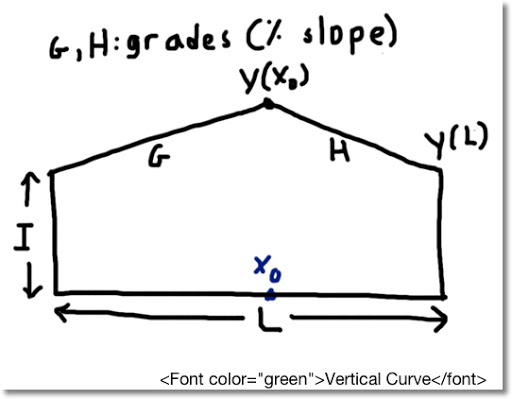## Sunday, May 19, 2013

### HP35S Vertical Curve: Elevation at Peak and at End PointHP35S Vertical Curve: Elevation at Peak and Length

Source: Fundamental of Engineering Supplied-Reference Handbook 8th Ed, 2nd Revision, 2011, NCEES

Calculator: HP 35S

Input
I = initial height
G = grade 1; entering the curve (in decimal form)
H = grade 2; exiting the curve (in decimal form)
L = horizontal length of the curve

G and H are the opposite signs

If G>0 and H<0 a="" and="" curve="" g="" h="" has="" if="" peak.="" similarity="" the="">0, the curve has a valley.

The program listed prompts for the inputs.

Output
1. Point where the curve reaches extreme elevation
2. Press R/S to get the elevation at the extreme point.
3. Press R/S once more to get the elevation at the end of the curve.

None of the outputs are stored.

Formula
A = (G - H)/(2L)
X_extrema = -G/(2A)
Equation of the Curve: y = I + G*x + A*x^2

Examples
Uphill curve:
I = 1,000 ft
G = 7% = 0.07
H = -4% = -0.04
L = 1,368 ft

Point at peak elevation is 870.545 ft into the curve at 1,030.469 ft. The elevation at the end of the curve is 1,020.520 ft.

Downhill curve:
I = 1,580 ft
G = -3% = -0.03
H = 4.2% = 0.042
L = 2.3 mi = 12,144 ft

Point at trough elevation occurs 5,060 ft into the curve at elevation 1,504.1 ft. The elevation at the end of the curve is at 1,652.864 ft.

Program
V001 LBL V
V002 INPUT I
V003 INPUT G
V004 INPUT H
V005 INPUT L
V006 RCL H
V007 RCL - G
V008 2
V009 ÷
V010 RCL ÷ L
V011 STO A
V012 RCL G
V013 x<>y
V014 ÷
V015 2
V016 ÷
V017 +/-
V018 R/S \\ shows point where extreme elevation occurs
V019 XEQ V024
V020 R/S \\ shows extreme elevation
V021 RCL L
V022 XEQ V024 \\ shows ending elevation
V023 RTN
V024 ENTER \\ calculate y subroutine
V025 RCL × A
V026 RCL + G
V027 ×
V028 RCL + I
V029 RTN

This blog is property of Edward Shore. 2013